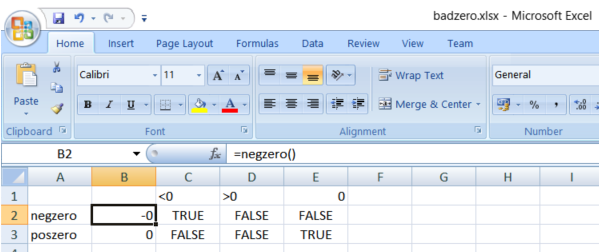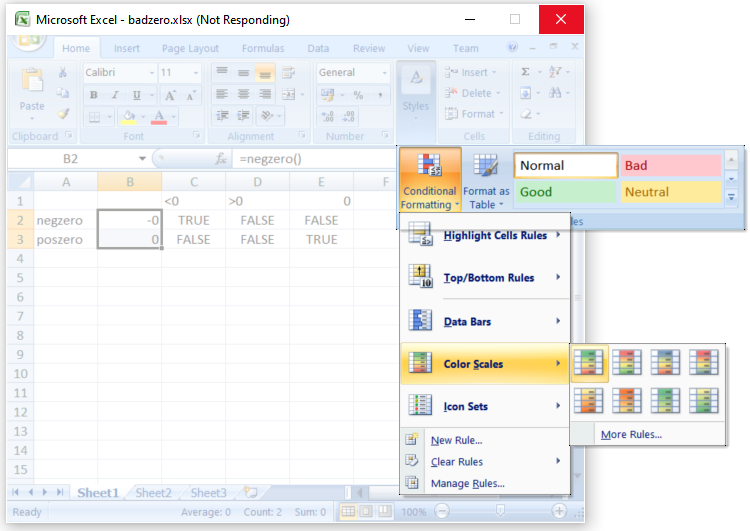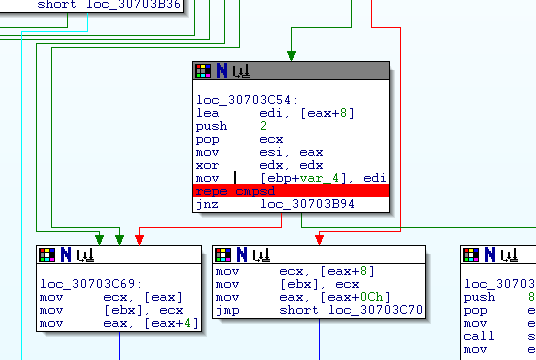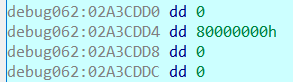benf.org :  excel stuff :  negative zero

## Overview

A colleague did a fabulous bit of grinding detective work, and tracked down a failure mode in which excel hangs. Here's a reproduction, and an explanation.

## Floating point, a reminder.

IEEE_754 specifies how we define floating point numbers.(Image from wikipedia)

We have a sign, an exponent and a mantissa. A number is (ignoring the detail of subnormal numbers) equal to (-1)s * 2e-1023 * 1.f

## So what's a zero?

Zero is defined by e and f both being all 0. However..... There are two zeros defined.

ValueHex
000000000 00000000
-080000000 00000000

In the case of -0, the top bit (i.e. the sign bit) is set.

## But excel can't do -0, can it?

Ordinarily, no. Excel normalizes away -0 to 0. We can't even persist it in a formula, really. However, I can show you how little excel cares using the wizard.but.... I can write an addin which returns negative zero...

Here's the (trivial) source, and here's a (32 bit) binary

We define two zeros - positive and negative

```extern "C" __declspec(dllexport) double poszero() { return 0.0; }
extern "C" __declspec(dllexport) double negzero() { return -0.0; }
```Excel will happily accept our -0, but something looks off. According to IEEE_754, negative and positive zero should compare equal.

## So what's the problem?

My colleague noticed that when you try to use some types of conditional formatting (eg colour scales) against -0, excel dies... horribly!They found this digging around on a recent version of 64 bit excel, but I've reproduced this on 32 bit Excel 2007. It's not new....

## Why?

At this point, we trot out IDA. Ida 5.0 is a free version, yay!

Since this is an infinite loop, we can just attach, and eyeball the threads.... In the relevant bit of code, when doing floating point operations - excel is performing floating point operations using the fp instruction set, and masking off to check results (here checking for infinity).Ok - seems reasonable... after a bit of digging, I found this:Here, excel is comparing two 8 byte values. Elsewhere, (i.e. in the snippet above) we take this value and cast it to a double, so we can tell that excel is considering these to be doubles.... what are they?Yup. We're using repe cmpsd (compare doubleword, twice) - comparing our positive zero against our negative zero, but using bitwise comparisons. Which will incorrectly say they aren't equal.

Now, we have a problem. These values are equal, according to floating point maths, but as 64 bit integers? No. Round the loop we go.... forever!

## Lessons learned

• Addin writers : When writing an addin, always return x == 0 ? 0 : x for doubles.
• Microsoft : DON'T TREAT DOUBLES AS LONGS, KIDS!

## Bonus level - sorting!

I expected this to only affect rendering, because that's a code path that doesn't (I imagine) get taken as much as standard calculation paths.
But what happens if we try to sort our zeros? We've already seen that -0 is apparently <0. So of course it will sort before, right?Wrong. Sorting isn't consistent with the (incorrect) comparisons we found earlier. (Though technically the sort is correct, given that -0.0 == 0.0..... ;) )

 Last updated 11/2020